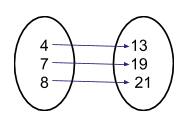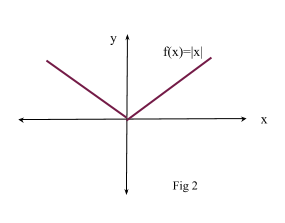# Functions

Contents

## Summary

• A function is defined as a rule which assigns each element of set X, one and only one element of set Y.  i.e set$X\quad =\quad \left\{ { x }_{ 1 },\quad x_{ 2 },\quad { x }_{ 3 } \right\}$  and set$Y\quad =\quad \left\{ { y }_{ 1 },\quad { y }_{ 2 },\quad { y }_{ 3 } \right\}$
• Domain: elements of set X
• Range: elements of set Y
• One to One Function: for each element of set Y, there is a unique corresponding element in set X.
• Many to One Function: for any element of set Y, there is more than one element in set X.
• Inverse Function:$f^{ -1 }\left( y \right) \quad =\quad x$
• Composite Function: combine two functions to get a new function$f\left[ g\left( x \right) \right]$
• Modulus Function:$f\left( x \right) \quad =\quad \left| x \right|$

Let’s suppose we have two sets of numbers:$X\quad =\quad \left\{ { 10,\quad 20,\quad 30,\quad 40 } \right\}$$Y\quad =\quad \left\{ { 32,\quad 62,\quad 92,\quad 122 } \right\}$

To define a relationship between these two sets we write a function:$f\left( x \right)\quad =\quad 3x\quad +\quad 2$ or can be written as$f\quad :\quad x\quad \rightarrow \quad 2x\quad +\quad 3$

Now we can see that for every value of set X, we have a value for set Y i.e

When$x\quad =\quad 10$$f\left( x \right)\quad =\quad 3(10)\quad +\quad 2\quad =\quad 32$

When$x\quad =\quad 20$$f\left( x \right) \quad =\quad 3(20)\quad +\quad 2\quad =\quad 62$   and so on…

If the following two conditions are satisfied than the relation in called a “Function”:

i) we can say that every element of X has an element in set Y.
ii) every element of X has only one unique element in set Y.

Therefore, a function is defined as a rule which assigns each element of set X, one and only one element of set Y.

#### Domain and range

Going back to the above example which consisted of two sets:$X\quad =\quad \left\{ { 10,\quad 20,\quad 30,\quad 40 } \right\}$$Y\quad =\quad \left\{ { 32,\quad 62,\quad 92,\quad 122 } \right\}$

Here elements of set X are known as “Domain” and elements of set Y are known as “Range”.

Hence we can write the domain and range as:

Domain of f = {10, 20, 30, 40}
Range of f = {32, 62, 92, 122}

Note: we cannot define a function if its domain is not mentioned, moreover range of a function may or may not consist of all elements of the set Y.

#### Example #1

Q. Let$f\quad :\quad x\quad \rightarrow \quad { (x\quad -\quad 4) }^{ 2 }\quad +\quad 8$  with domain x > 0.

Find the range of f.

Solution:

Since a function consists of a square, and we know that result of a square is always positive, therefore all values of f(x) will be greater than 8.$f\quad :\quad x\quad \rightarrow \quad { (positive\quad value) }\quad +\quad 8$

Hence the range of f is  > 8

#### One to One Function

If we have a function f such that for each element of set Y (the range), there is a unique corresponding element in set X (the domain), then f is called a one to one function i.e:

given$f\left( x \right)\quad =\quad 2x\quad +\quad 5$ from X to Y where X = {4, 7, 8} is one to one as shown below:#### Many to One Function

If we have a function f such that for any element of set Y (the range), there is more than one element in set X (the domain), then f is called a many to one function, i.e:$f\quad :\quad x\quad \rightarrow \quad 2{ x }^{ 2 }\quad +\quad 4x\quad -6$ is a many to one function as the result x = 1 & -3 both correspond to 0 of set Y.

#### Composite Functions

Suppose we have two functions:$f\quad :\quad x\quad \rightarrow \quad { x }^{ 2 }$   and$g\quad :\quad x\quad \rightarrow \quad { 2x\quad +\quad 3 }$

We can combine these two functions to get a new function$f\left[ g\left( x \right) \right]$, and this new function is known as a composite function, i.e:

for the above two functions$f\left[ g\left( x \right) \right]$ means:$=\quad f\left[ g\left( x \right) \right]$$=\quad f\left( 2x\quad +\quad 3 \right)$

Since:$f\left( x \right) \quad =\quad { x }^{ 2 }$$f\left[ g\left( x \right) \right] ={ (2x\quad +\quad 3) }^{ 2 }$

#### Inverse Function

Suppose we have a function$f\left( x \right)\quad =\quad y$, inverse of this function is the one that uses reverse relation i.e$f^{ -1 }\left( x \right) \quad =\quad y$

However, remember that an inverse of a function will only exist if a function is one to one. Furthermore, if we draw the graphs of$f\quad and\quad { f }^{ -1 }$ on the same axis, then the graph of${ f }^{ -1 }$ will be the reflection of f in the line y = x as shown in Fig 1#### Example #2

Q.$f\quad :\quad x\quad \rightarrow \quad \frac { 3x\quad +\quad 4 }{ 2 } ,\quad find\quad { f }^{ -1 }$

Solution:

We know:$f\quad :\quad x\quad \rightarrow \quad \frac { 3x\quad +\quad 4 }{ 2 }$$y\quad =\quad \frac { 3x\quad +\quad 4 }{ 2 }$

To find inverse make x the subject of equation:$2y\quad =\quad 3x\quad +\quad 4$$2y\quad -\quad 4\quad =\quad 3x$$x\quad =\quad \frac { 2y\quad -\quad 4 }{ 3 }$

Hence,${ f }^{ -1 }\quad \rightarrow \quad x\quad =\quad \frac { 2y\quad -\quad 4 }{ 3 }$      Ans

#### Modulus Function

A modulus is defined as an absolute value of x. It is basically a distance of x from a fixed point. It is written as$\left| x \right|$. A modulus function is defined as:$f\left( x \right)\quad =\quad \left| x \right|$$\left| x \right| \quad =\quad x\quad for\quad x\quad >\quad 0\quad and\quad \left| x \right| \quad =\quad -x\quad for\quad x\quad <\quad 0$

Therefore the graph of$y\quad =\quad \left| x \right|$
is line y = x for x > 0 and y =  -x for x < 0 as shown below in Fig 2:#### Graphical Transformations

Suppose we have a function$y\quad =\quad f\left( x \right)$, some rules to remember when transforming graphs are:

• The graph of$y\quad =\quad f\left( x \right) \quad +\quad c$ where c is a constant, has the same shape of the function$f\left( x \right)$ but is moved c units higher.
• The graph of$y\quad =\quad f\left( x \right) \quad -\quad c$ where c is a constant, has the same shape of the function$f\left( x \right)$ but is moved c units lower.
• The graph of$y\quad =\quad f\left( x\quad +\quad k \right)$, where k is a constant, has the same shape of the function but is moved k units to the left.
• The graph of$y\quad =\quad f\left( x\quad -\quad k \right)$, where k is a constant, has the same shape of the function but is moved k units to the right.
• The graph of$y\quad =\quad f\left( -x \right)$ is simply a reflection of$f\left( x \right)$  in the y axis.
• The graph of$y\quad =\quad -f\left( x \right)$ is a reflection of$f\left( x \right)$ in the x axis.
• The graph of$y\quad =\quad a\quad f\left( x \right)$ is stretched by a scale factor a in the y axis.
• The graph of$y\quad =\quad f\left( ax \right)$ is stretched by a scale factor$\frac { 1 }{ a }$ in the x axis.